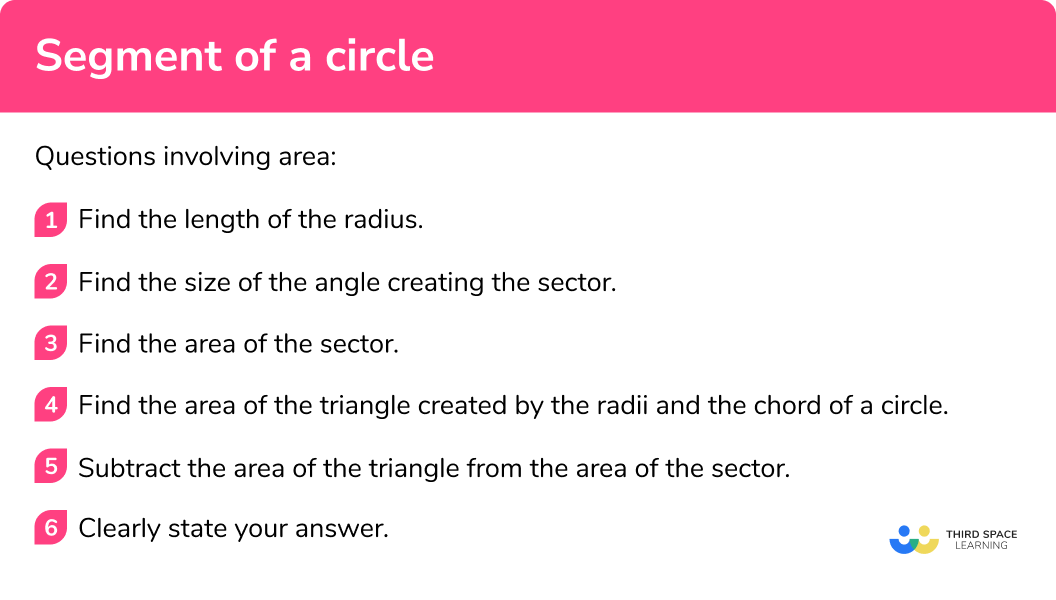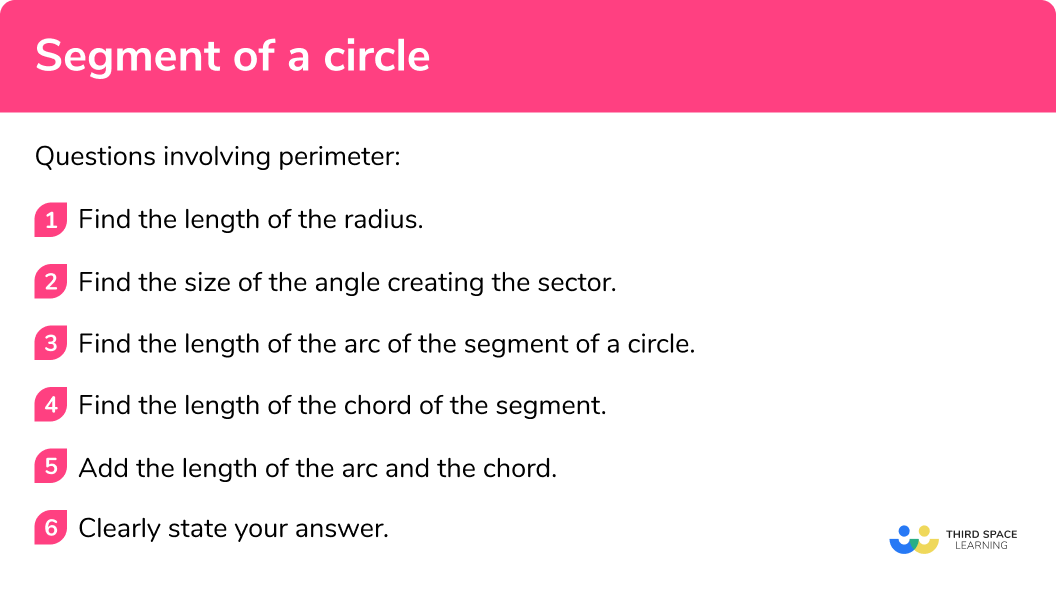# Segment Of A Circle

Here we will learn about the segment of a circle including how to identify the segment of a circle and how to find the area of a segment given the different parts of a circle.

There are also parts of a circle worksheets based on Edexcel, AQA and OCR exam questions, along with further guidance on where to go next if you’re still stuck.

## What is the segment of a circle?

A segment of a circle is the area enclosed by an arc of a circle and a chord.

There are two main types of segment:

• The major segment is the segment where the arc length is greater than half the circumference of the circle
• The minor segment is the segment where the arc length is less than half the circumference of the circle

An arc is a fraction of the circumference of a circle.

A chord is a line segment that connects two points of a circle.

A segment where the chord passes through the centre of the circle is called a semicircle.

### What is the segment of a circle?## How to solve problems involving a segment of a circle

In order to solve problems involving a segment of a circle:

Questions involving area

1. Find the length of the radius.
2. Find the size of the angle creating the sector.
3. Find the area of the sector.
4. Find the area of the triangle created by the radii and the chord of a circle.
5. Subtract the area of the triangle from the area of the sector.

### How to solve problems involving a segment of a circle (area)Questions involving perimeter

1. Find the length of the radius.
2. Find the size of the angle creating the sector.
3. Find the length of the arc of the segment of a circle.
4. Find the length of the chord of the segment.
5. Add the length of the arc and the chord.

### How to solve problems involving a segment of a circle (perimeter)Segment of a circle is part of our series of lessons to support revision on circles, sectors and arcs. You may find it helpful to start with the main circles, sectors and arcs lesson for a summary of what to expect, or use the step by step guides below for further detail on individual topics. Other lessons in this series include:

## Segment of a circle examples

### Example 1: calculate the area of a segment of a circle

Calculate the area of the segment shown below. Give your answer to 3 significant figures

1. Find the length of the radius.

Length of the radius of the circle: 7cm

2Find the size of the angle creating the sector.

The size of the angle creating the sector (made by the two radii) is 90^{\circ} .

3Find the area of the sector.

$\text{Area of sector }=\frac{\theta}{360} \times \pi r^{2}\\ =\frac{90}{360} \times \pi \times 7^{2} \\ =\frac{49}{4} \pi$

Area of sector: \frac{49}{4} \pi \mathrm{cm}^{2}

It is important to not round the answer at this stage of the question

4Find the area of the triangle created by the radii and the chord.

$\text{Area of triangle} = \frac{1}{2} a b \sin C \\ =\frac{1}{2} \times 7 \times 7 \times \sin(90)\\ =24.5$

Area of triangle: 24.5cm^{2}

5Subtract the area of the triangle from the area of the sector.

$\text{Area of sector – Area of triangle} = \frac{49}{4} \pi-24.5\\ =13.9845…$

Area of the segment of the circle= 13.9845...cm^{2}=14.0cm^{2} ( 3 .s.f.)

### Example 2: calculate the perimeter of a segment

Calculate the perimeter of the segment shown below. Give your answer to 3 decimal places

Find the length of the radius.

Find the size of the angle creating the sector.

Find the length of the arc of the segment.

Find the length of the chord of the segment.

Add the length of the arc and the chord.

### Example 3: calculate the area of a segment given the length of the radii and the angle

Calculate the area of the segment shown below. Give your answer to 3 decimal places

Find the length of the radius.

Find the size of the angle creating the sector.

Find the area of the sector.

Find the area of the triangle created by the radii and the chord.

Subtract the area of the triangle from the area of the sector.

### Example 4: calculate the perimeter of a segment given the length of the radii and the angle

Calculate the perimeter of the segment shown below. Give your answer to 3 decimal places.

Find the length of the radius.

Find the size of the angle creating the sector.

Find the length of the arc of the segment of a circle.

Find the length of the chord of the segment.

Add the length of the arc and the chord.

### Example 5: calculate the area of a segment without the angle of the segment being given

Calculate the area of the segment shown below. Give your answer to 1 decimal place

Find the length of the radius.

Find the size of the angle creating the sector.

Find the area of the sector.

Find the area of the triangle created by the radii and the chord.

Subtract the area of the triangle from the area of the sector.

### Example 6: calculate the perimeter of a segment without the angle of the segment being given

Calculate the perimeter of the segment shown below. Give your answer to 1 decimal place

Find the length of the radius.

Find the size of the angle creating the sector.

Find the length of the arc of the segment.

Find the length of the chord of the segment.

Add the length of the arc and the chord.

### Common misconceptions

• Confusing the segment/sector

A segment is the area enclosed by the arc of a circle and a chord. A sector is the area enclosed by the arc of a circle and two radii.

• Confusing the chord/diameter

A chord can cross a circle at any point, but a diameter but go through the centre of the circle.

• Incorrect use of the cosine rule

Many mistakes are made when applying other rules within a segment question e.g the cosine rule. Take your time with these parts and regularly check that your answer makes sense within the context of the question.

• Not including the chord length when finding the perimeter of a segment

Remember the perimeter of a shape is the sum of the lengths of each of the sides. Therefore the perimeter of a segment is made up the arc and the chord

Angles can be measured in both degrees and radians, however at GCSE we only use degrees.

### Practice segment of a circle questions

1. What is the area of the segment in the diagram? Give your answer to 1 decimal place36\pi \mathrm{cm}^{2}72 cm^{2}41.1 cm^{2}41.097 cm^{2}\text{Area of sector }=\frac{\theta}{360} \pi r^{2}\\ =\frac{90}{360}\times \pi \times 12^{2}\\ =36 \pi

\text{Area of triangle }=\frac{1}{2}ab \sin C\\ =\frac{1}{2}\times 12 \times 12 \sin(90)\\ =72

\text{Area of segment }=36 \pi – 72\\ =41.09733553\\ =41.1\mathrm{cm}^{2}

2. What is the perimeter of the segment in the diagram? Give your answer to 1 decimal place12\pi40.97 cm20.7cm35.8 cm\text{Arc length}=\frac{\theta}{360} \times 2 \pi r \\ =\frac{90}{360} \times 2 \pi \times 12 \\ =6 \pi

Length of cord:

a^{2}+b^{2}=c^{2} \\ 12^{2}+12^{2}=c^{2} \\ 144+144=c^{2} \\ 288=c^{2} \\ 12 \sqrt{2}=c

\text{Length of the arc }+\text{ Length of the chord } = 6\pi + 12 \sqrt{2}\\ =35.82011867\\ =35.8\mathrm{cm}

3. Calculate the area of the segment shown. Give your answer to 1dp .22.3 m^{2}19.6m^{2}25.1m^{2}15.9m^{2}\text{Area of sector }=\frac{\theta}{360} \pi r^{2}\\ =\frac{160}{360}\times \pi \times 4^{2}\\ =\frac{64}{9} \pi

\text{Area of triangle }=\frac{1}{2}ab \sin C\\ =\frac{1}{2}\times 4 \times 4 \sin(160)\\ =2.73616…

\text{Area of segment }=\frac{64}{9} \pi – 2.73616\\ =19.60405\\ =19.6\mathrm{m}^{2}

4. Calculate the perimeter of the segment shown. Give your answer to 3 significant figures.1.92 m3.767 m1.85 m3.77 m\text{Arc length}=\frac{\theta}{360} \times 2 \pi r \\ =\frac{55}{360} \times 2 \pi \times 2 \\ =\frac{11}{18} \pi

Length of cord:

a^{2}=b^{2} + c^{2} -2bc \cos(A)\\ a^{2}=2^{2} + 2^{2} – 2 \times 2 \times 2 \times \cos(55)\\ a^{2}=8-8 \cos(55)\\ a^{2}=3.411388509\\ a=1.846994453

\text{Length of the arc }+\text{ Length of the chord } =\frac{11}{18} \pi + 1.846994453\\ =3.76685663

5. Calculate the area of the segment shown. Give your answer to 2 decimal places.14.21cm^{2}25.40cm^211.19cm^{2}18.66cm^{2}First we need to find the angle using the cosine rule.

\cos{A}=\frac{b^{2}+c^{2}-a^{2}}{2 b c} \\ \cos{A}=\frac{5^{2}+5^{2}-8.5^{2}}{2 \times 5 \times 5} \\ \cos{A}=-\frac{89}{200} \\ A=\cos^{-1}\left(-\frac{89}{200}\right) \\ A=116.4233388^{\circ}

\text{Area of sector }=\frac{\theta}{360} \pi r^{2}\\ =\frac{116.423}{360}\times \pi \times 5^{2}\\ =25.39955844

\text{Area of triangle }=\frac{1}{2}ab \sin C\\ =\frac{1}{2}\times 5 \times 5 \sin(116.423)\\ =11.194165

\text{Area of segment }=25.39955844 – 11.194165\\ =14.20539344\\ =14.21\mathrm{cm}^{2}

6. Calculate the perimeter of the segment shown. Give your answer to 3 significant figures.22.0mm16.5mm8.52mm22.5mmFirst we need to find the angle using the cosine rule.

\cos{A}=\frac{b^{2}+c^{2}-a^{2}}{2 b c} \\ \cos{A}=\frac{7^{2}+7^{2}-8^{2}}{2 \times 7 \times 7} \\ \cos{A}=\frac{17}{49} \\ A=\cos^{-1}\left(\frac{17}{49}\right) \\ A=69.69980^{\circ}

\text{Arc length}=\frac{\theta}{360} \times 2 \pi r \\ =\frac{69.6998}{360} \times 2 \pi \times 7 \\ =8.515436986

Chord length: 8mm

\text{Perimeter }=8.515436986+8\\ =16.515436986\\ =16.5\mathrm{mm}

### Segment of a circle GCSE questions

1. The diagram shows a sector OABC of a circle with centre O .(a) Calculate the length of the arc ABC of the sector. Give your answer correct to 3 significant figures.

(b) Calculate the area of the shaded segment ABC . Give your answer correct to 3 significant figures.

(7 marks)

(a)

\frac{120}{360} \times \pi \times 20.8

(1)

21.781709

(1)

21.8 cm

(1)

(b)

Either 113.26… or 46.83 seen

(1)

“113.26…” – “46.83”

(1)

66.430…

(1)

66.4 cm^{2}

(1)

2. The diagram shows a sector OPRS of a circle with centre O .Calculate the area of the shaded segment PRS .

(5 marks)

Area of sector = 22.34

(1)

Area of triangle = 20.569

(1)

“22.340…” – “20.569”

(1)

=1.7710…

(1)

=1.77cm^{2}

(1)

3. The diagram shows a sector OPRS of a circle with centre O .Calculate the area of the shaded segment RST .

(5 marks)

Area of sector = 1954.76876…

(1)

Area of triangle = 1835.444…

(1)

“1954.768…” – “1835.444”

(1)

119.324…

(1)

119cm^{2}

(1)

4. The diagram below shows a shaded segment.(9 marks)

(a)

Angle at centre = 112.88538 … may be shown on diagram

(1)

Area of sector = 141.85595…

(1)

Area of triangle = 66.332496…

(1)

“141.85595” – “66.332496”

(1)

= 75.523454..

(1)

75.5cm^{2}

(1)

(b)

Length of arc = 23.6426587…

(1)

Length of chord = 20

(1)

“23.6426587” + 20

(1)

= 43.6426587

(1)

43.6cm

(1)

## Learning checklist

You have now learned how to:

• Identify and apply circle definitions and properties
• Identify and apply circle definitions and properties, including a segment
• Find the perimeter of a segment
• Calculate areas of segments by applying trigonometry

## Still stuck?

Prepare your KS4 students for maths GCSEs success with Third Space Learning. Weekly online one to one GCSE maths revision lessons delivered by expert maths tutors.

Find out more about our GCSE maths tuition programme.